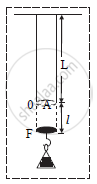HSC Science (Electronics) 12th Board ExamMaharashtra State Board
Share

# Within the Elastic Limit, Find the Work Done by a Stretching Force on a Wire. - HSC Science (Electronics) 12th Board Exam - Physics

#### Question

Within the elastic limit, find the work done by a stretching force on a wire.

#### Solution

a.

Let,
L = length of wire
A = area of cross section of wire
r = radius of cross section of wire
l = elongation of the wire by applying load.

b.

If the wire is perfectly elastic then,
Young’s modulus,Y=(F/A)/(l/L)

=F/AxxL/l

F=(YAl)/L..............(1)

c.   Let ‘f’ be the restoring force and ‘x’ be its corresponding extension at certain instant during the process of extension.

f=(YAx)/L................(2)

d.  Let ‘dW’ be the work done for the further small extension ‘dx’.

dW=fdx

dW=(YAx)/Ldx ..........(3)

e.  The total amount of work done in stretching the wire from 0 to l can be found out by integrating equation (3).

W=int_0^ldW=int_0^l(YAx)/Ldx=(YA)/L int_0^lx dx

W=(YA)/L[x^2/2]_0^l

W=(YAl^2)/2L

W=(YAl)/L.l/2

But,(YAl)/L=F

therefore W=1/2xxfxxl ..................(4)

Equation (4) represents the work done by stretching a wire.

Is there an error in this question or solution?

#### APPEARS IN

2016-2017 (July) (with solutions)
Question 2.2 | 3.00 marks

#### Video TutorialsVIEW ALL 

Solution Within the Elastic Limit, Find the Work Done by a Stretching Force on a Wire. Concept: Elastic Energy.
S